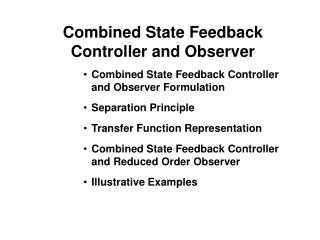DownloadDownload PresentationCombined State Feedback Controller and Observer

# Combined State Feedback Controller and Observer

Télécharger la présentation## Combined State Feedback Controller and Observer

- - - - - - - - - - - - - - - - - - - - - - - - - - - E N D - - - - - - - - - - - - - - - - - - - - - - - - - - -
##### Presentation Transcript

1. Combined State Feedback Controller and Observer • Combined State Feedback Controller and Observer Formulation • Separation Principle • Transfer Function Representation • Combined State Feedback Controller and Reduced Order Observer • Illustrative Examples

2. Motivation • In most control applications all state variables are not measurable • A full or reduced order observer may be used to estimate needed states • Separation principle allows independent controller and observer design

3. Combined Controller-Observer Formulation Plant: Controller: Observer:

4. State Feedback Observer Block Diagram u y r System L x y z-1 C H Observer G -K

5. Closed-Loop System Closed-Loop Control Subsystem: Closed-Loop Observer Subsystem: Overall Closed-Loop System:

6. Separation Principle Eigenvalues of the closed loop systems are the union of eigenvalues of • Closed-loop poles, i.e., eigenvalues of G-HK and • Observer poles GA-LC

7. Separation Principle--Transfer Function Closed-Loop System Laplace Transform: Closed-loop transfer function • Closed-loop transfer function is the same as full state feedback • Observer dynamics are canceled

8. Laplace Domain Representation Plant: Controller: Observer: Laplace Transform of Observer Controller in z-domain:

9. Transfer Function Block Digaram Representation Control Input: Solving for U gives where Y R G F1 F2

10. D.C. Motor Example Consider a d.c. servomotor system given by the transfer function y: position output u: input voltage the motor. Motor parameters: k/J=1 and b/J=5.

11. Feedback Control Design for D.C. Motor Example Desired Closed-Loop System: damping ratio =0.8 and natural frequency n=500 rad/sec (less than 3% maximum overshoot and settling time of 0.01 sec.): System in Controllable Form: Control gain

12. Servo Example Observer Design Observer Dynamics (2 times faster than controller): =0.8 and n=1000 rad/sec Observer gain: Observer State Equations

13. Equivalent Transfer Function of Servo Example Feedback and Feedforward Block: where

14. Closed-Loop Transfer Function R G F1 F2 Pole-zero Cancellation

15. Combined Controller Reduced Order Observer (CCRO) Plant State Equation: Reduced Order Observer

16. Transfer Function Representation of CCRO Partition State Feedback Gain: Reduced Order Observer Transfer Function Substitute Z in Control Law: where

17. Transfer Function Block Digaram Representation of CCRO Y R G F1 F2 F1 and F2 are of order n-q (lower than FOO)

18. D.C. Motor Example

19. Closed-Loop System of CCRO Example

20. Matlab Solution %Simulation Example of Combined Observer %System: G(s)=b/(s^2+bs) % Observable form: dx1/dt=x2, dx2/dt=-ax2+bu %System Matrices b=1; a=5; A=[0 1;0 -a]; B=[0;b]; C=[1 0]; D=0; plant=ss(A,B,C,D);

21. MATLAB Example Control Design %Control Design %desired closed-loop damping and natural frequency zeta=0.8; wn=500; pd=-zeta*wn+sqrt(zeta^2-1)*wn; %desired closed-loop poles %Ackermans's formula to find gain K=acker(A,B,[pd;conj(pd)]);

22. MATLAB Example FOO Design %Full-order observer r=2; pdo=-r*zeta*wn+r*sqrt(zeta^2-1)*wn; %desired observer poles L=(acker(A',C',[pdo;conj(pdo)]))'; Ao=A-L*C; ff=ss(Ao,B,K,0); %Part of feedforward block fb=ss(Ao,L,K,0); %Feedback Block g=-(K/Ao)*L;

23. MATLAB Example ROO Design %Reduced-order observer pr=-r*wn; A11=A(1,1); A12=A(1,2); B1=B(1); A21=A(2,1); A22=A(2,2); B2=B(2); K1=K(1); K2=K(2); Lr=(A22-pr)/A12; Ar=A22-Lr*A12; By=A21-Lr*A11+Ar*Lr; Bu=B2-Lr*B1; ffr=ss(Ar,Bu,K2,0); fbr=ss(Ar,By,K2,K1+K2*Lr); gr=-(K2/Ar)*By+K1+K2*Lr;